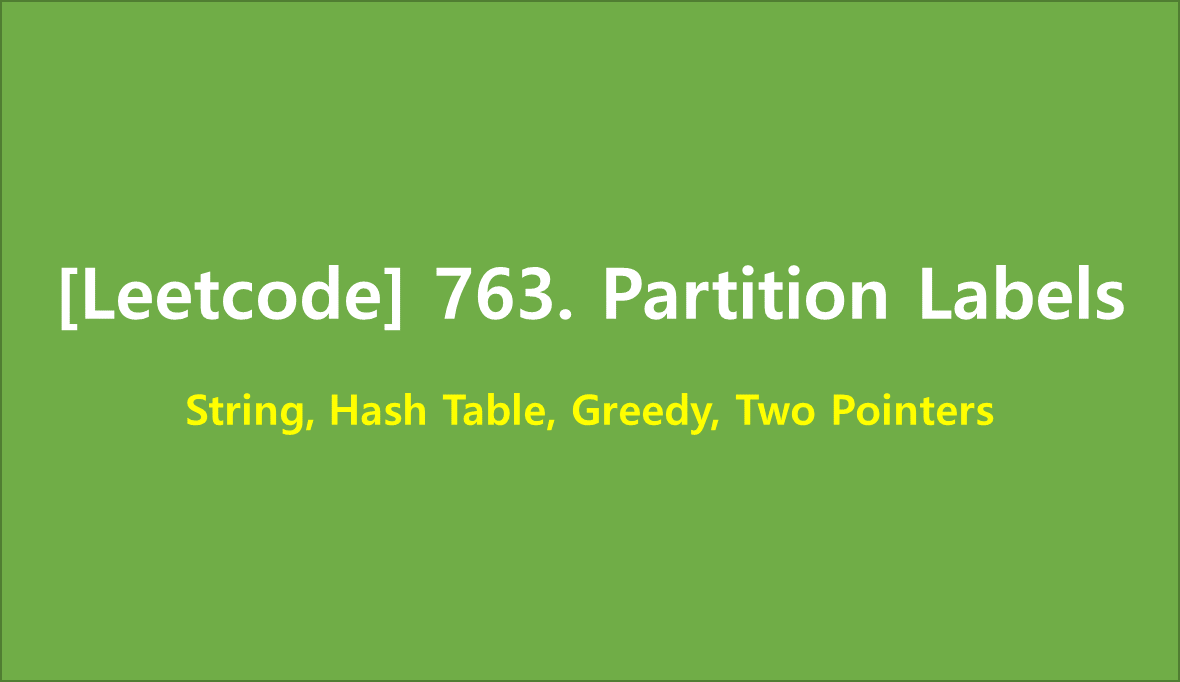# [Leetcode] 763. Partition Labels

limelimejiwon·2022년 5월 18일
0

## Python으로 공부하는 코딩테스트

목록 보기
52/67## 📄 Description

You are given a string s. We want to partition the string into as many parts as possible so that each letter appears in at most one part.

Note that the partition is done so that after concatenating all the parts in order, the resultant string should be s.

Return a list of integers representing the size of these parts.

### Example 1:

Input: s = "ababcbacadefegdehijhklij"
Output: [9,7,8]
Explanation:
The partition is "ababcbaca", "defegde", "hijhklij".
This is a partition so that each letter appears in at most one part.
A partition like "ababcbacadefegde", "hijhklij" is incorrect, because it splits s into less parts.

### Example 2:

Input: s = "eccbbbbdec"
Output: 

### Constraints:

• 1 <= s.length <= 500
• s consists of lowercase English letters.

## 💻 My Submission

class Solution:
def partitionLabels(self, s: str) -> List[int]:
_dict=defaultdict(list)
# check out the partition
for index,alpha in enumerate(s):
if len(_dict[alpha])<2:
_dict[alpha]=[index,index]
else:
_dict[alpha][-1]=index

# make partition Lables
for s,e in list(_dict.values()):

return [e-s+1 for s,e in answer]

## 🕐Complexity

### 📌 Time Complexity: O(n)

• n is the size of string s,
• O(m) for the size of set(s)
• n>=m, so as a result, Time complexity will be O(n)

### 📌 Space Complexity: O(1)

• hashmap consist of max 26 keys

## 🎈 Better Solution

class Solution:
def partitionLabels(self, s: str) -> List[int]:
L = len(s)
last = {s[i]: i for i in range(L)} # last appearance of the letter
i, ans = 0, []
while i < L:
end, j = last[s[i]], i + 1
while j < end: # validation of the part [i, end]
if last[s[j]] > end:
end = last[s[j]] # extend the part
j += 1

ans.append(end - i + 1)
i = end + 1

return ans

References